Tapping into mathematics

Start this free course now. Just create an account and sign in. Enrol and complete the course for a free statement of participation or digital badge if available.

Free course

# 3 Some calculator puzzles

If you would like some more calculator practice, try your hand at the following puzzles. No answers are given because most of these activities have no single numerical answer. You may like to try them out with your friends – or make up some of your own.

## Brain stretcher: Doing it with your eyes closed!

Look at your calculator for about one minute. Now turn it over and see how many of the keys you can draw in their correct position.

• Why are the keys laid out as they are?

• Can you classify the keys into four or five useful headings?

## Brain stretcher: Is it true?

Here are some mathematical statements for you to explore with your calculator. Are they true? If not, why not? Can you modify them so that they are true?

• You can't take bigger from smaller.

• Multiplying makes numbers bigger.

• The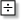key is the ‘divide into’ key.

• The more digits a number has, the bigger it is.

• There are two ‘minus’ keys, one marked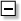and the other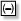## Brain stretcher: Missing keys

Can you make all the numbers from 0 to 9 using just these keys?

4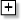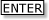You can use any of them more than once and you need not use them all in a given sequence.

Write down key sequence for each number, trying to use as few key presses as possible.

For example. 5 could be 444.

## Brain stretcher: Big sum, small sum

Use the digits 1, 2, 3, 4, and 5 just once in any order to form two numbers; for example, 123 and 54.

Which numbers have:

• the greatest sum?

• the least sum?

• the greatest difference?

• the least difference?

## Brain stretcher: Big product, small product

Use the digits 1, 2, 3, 4 and 5 just once to form two numbers so that:

• their product is greatest

• their product is least (the product of two numbers is what you get when they are multiplied together).

Try extending the problem by using six digits, seven digits and so on.

## Brain stretcher: 1089 and all that

Take a three-digit number, say 417.

Reverse the digits and subtract whichever number is the smaller from the bigger:

714 − 417 = 297

792 + 297 = 1089

Try this process for other three-digit numbers. Do you always get 1089? Which numbers does it not work for?

What if…?

## Brain stretcher: Puzzling behaviour

Type any number in your calculator and press.

Now press52Keep on pressingmany times

What is going on?

Try other starting numbers.

Try changing the 5 and/or the 2.

MU120_4MCAL